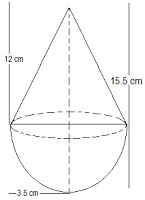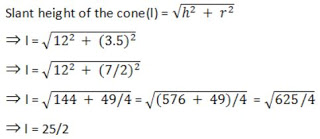Newbie

# A toy is in the form of a cone of radius 3.5 cm mounted on a hemisphere of same radius. The total height of the toy is 15.5 cm. Find the total surface area of the toy.Q.3

• 0

Find the best method of the ncert class 10 chapter surface areas and volumes . In exercise 13.1 question number 3 is very tricky question i don’t understand the formula of this question please help me put the formula into the question also  give the best solution of the question. A toy is in the form of a cone of radius 3.5 cm mounted on a hemisphere of same radius. The total height of the toy is 15.5 cm. Find the total surface area of the toy.

Share

1. The diagram is as follows:Given that the radius of the cone and the hemisphere (r) = 3.5 cm or 7/2 cm

The total height of the toy is given as 15.5 cm.

So, the height of the cone (h) = 15.5-3.5 = 12 cm∴ The curved surface area of cone = πrl

(22/7)×(7/2)×(25/2) = 275/2 cm2

Also, the curved surface area of the hemisphere = 2πr2

2×(22/7)×(7/2)2

= 77 cm2

Now, the Total surface area of the toy = CSA of cone + CSA of hemisphere

= (275/2)+77 cm2

= (275+154)/2 cm2

= 429/2 cm2 = 214.5cm2

So, the total surface area (TSA) of the toy is 214.5cm2

• 0